## Wednesday, December 27, 2017

Unit – 4: The Theory of firm under Perfect Competition and Equilibrium
1. What do you mean by Market in general?
Ans: In general sense, market means a place where commodities are bought and sold.
2. What is market in Economics?
Ans: In economics, market means a group of buyers and sellers exchanging a particular commodity. Thus market means the market for a commodity where it indicates all transactions connected with purchase and sale of a commodity.
3. What do you mean by perfect competition?
Ans. Perfect competition refers to a market situation in which there are large numbers of buyers and sellers. They produced homogeneous product at the given market price.
4. What are specific features of Perfectly competitive market? 2012
Ans: the goods are sold of uniform price, products are homogeneous and the average and marginal revenue of a firm always equal.
5. In the perfectly competitive market a firm attains equilibrium at that point of P = MC =____.
Ans. MR.
6. What is Shut-down point? 2013, 2015

Ans: A firm will not produce at a price which is lower than the average variable cost. So long price is above the average variable cost, production will go on. If the price falls below the average variables cost, it will stop production. It is the Shut-down point. Thus in the short run a firm will continue to produce till its price covers the average variable cost of a firm.
7. Under a perfectly competitive market each buyer and seller is a ____. Ans. Price taker
8. For a price taking firm (Perfectly Competitive market), marginal revenue equals the market price. Ans. True
9. The difference between total revenue and total cost indicates the amount of profit for the firm. Ans. True
10. The demand curve that a firm faces in a perfectly competitive market perfectly inelastic.  Ans. False
11. Buyers have perfect information about the price prevailing in the market. Ans. True
12. Profit that a firm earns over and above the normal profit is called the ____.  Ans. Supernormal profit
13. The point on the supply curve at which a firm earns normal profit is called ____. Ans. Breakeven point
14. At the point of equilibrium of a firm under perfect competition P = AC = ____. Ans. MC
15. TR = P (Price) X ____. Ans. Q (quantity)
16. R (Profit) = TR _____. Ans. TC
17. Under Perfectly competitive market who is the price taker ____. Ans. Seller.
18. For a price taking firm, average revenue is equal to ____. Ans. Marginal revenue 2013
19. All firms under perfect competition produce ____.  Ans. Homogeneous
20. Draw the average revenue and marginal revenue curve of a perfectly competitive firm.
Ans: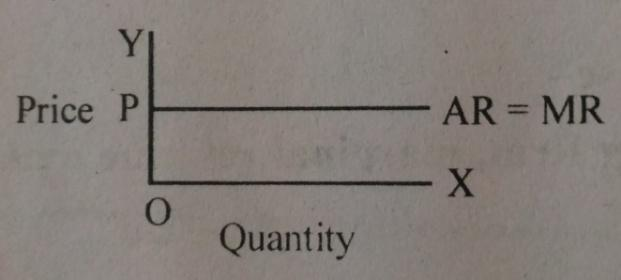21. What do you mean by price curve of perfectly competitive market?
Ans: The demand curve of a perfectly competitive market is called price curve.
22. Give the meaning of equilibrium price. Or Define equilibrium price. 2015
Ans: The price which equates market demand of a commodity with its market supply is the equilibrium price. Equilibrium Price: Market Demand = Market Supply.
23. Who determines price under perfect competition?
Ans: Price under perfect competition is determined by the forces of market demand and market supply.
24. Give the meaning of excess demand for a product.
Ans: When market demand exceeds market supply of a commodity at a given price it is known as excess demand.
25. Give the meaning of excess supply of a product.
Ans: Excess supply means market supply of a commodity is more than market demand for a commodity at the given price.
26. What is market period?
Ans. The market period is very short period in which supply is fixed.
27. What is market price?
Ans. The market price is very short period price like an hour, a day or few days. Demand plays an active role for determining price in such a market.
28. What is normal price?
Ans. The normal price is that price in which time is available to adjust the demand with the supply.
29. What is long run price?
Ans. Long run price is known as normal price. It is determined by long run equilibrium between demand and supply.
30. What is short period?
Ans. Short period means that period in which time is not available to change its demand to its supply
31. What is reserve price?
Ans. The minimum price at which the sellers refuse to supply the good at all and store it with themselves is known as reserve price.
32. What is normal profit?
Ans. Normal profit is that which is just sufficient to induce the individual firms to remain in the industry. In that situation total revenue is equal to total cost.
33. What is Super-normal profit?
Ans. Super-normal profit is that profit, in which the total revenue is higher than its total cost.
34. What are the factors that determine the reserve price?
Ans. Following are the factors that determine the reserve price as follows:
1. The durability of the good is one of the important factors that determine the reserve price.
2. Production cost to be incurred in the future on a good governs the reserve price.
3. Another factor which determines the reserve price is the sellers preference for liquidity.
35. Define market equilibrium.
Ans. Market equilibrium is a situation of zero excess demand and zero excess supply. Or it is a situation where: market demand = market supply.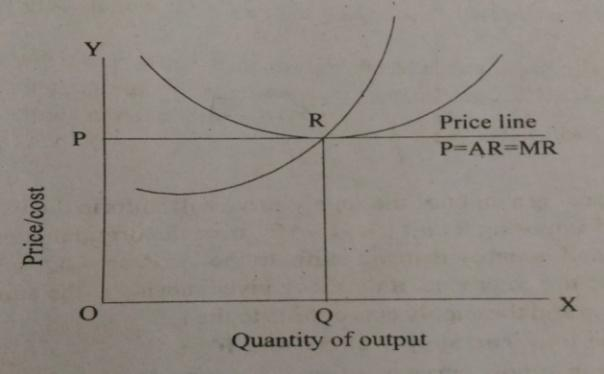-Market Equilibrium: -Excess Supply: -Excess Demand: Market Supply = Market Demand Market Supply > Market Demand Market Demand > Market Supply

36. Write four features of perfect competition. 2014
Ans: Characteristics or Features of Perfect competition are as follows:-
1. Large numbers of Buyers and Sellers: The number of buyers and sellers of a commodity is very large under perfect competition but no seller or buyer can influence the price.
2. Homogeneous Product: All sellers sell identical units of a given product.
3. Perfect Knowledge: Buyers and sellers have full knowledge regarding the prevailing market price.
4. Freedom of Entry and Exit: A firm can enter or leave the industry any time. There are no restrictions.
5. Perfect Mobility: Factors of production are perfectly mobile.
6. Lack of Selling Cost: The sellers do not spend on advertisement and publicity.
37. Why is a firm under perfect competition a price taken?
Ans: A firm under perfect competition a price taken by the following reasons:-
1. Number of firms: The number of firm under perfect competition is so large that no individual firm by change sale, can cause any meaningful change in total market supply. Hence market price remains unaffected.
2. Homogeneous product: All firm in a perfectly competitive produce homogeneous product. Hence price remains same.
3. Perfect Knowledge: All the buyers and sellers have perfect knowledge about market price so no firm charge a different price than market price. Hence, a uniform price prevails in the market.
38. Define market and explain briefly four basis on which different markets are defined.
Ans. Markets refers to the mechanism of sale and purchase of commodities. Following factors determine different market structures:-
1. Number of Firms Operating in the Industry: If there are a large number of firms in the industry, the market is either perfect competition or monopolistic competition. Only one firm in the market for a commodity implies monopoly. Existence of a few firms implies oligopoly.
2. Nature of Product: Homogeneous product is found in perfect competition or even in case monopoly. Product differentiation is the characteristic feature of monopolistic competition. However monopolist may also practice product differentiation.
3. Control Over Price: If an individual producer in the market has no control over price, it is a situation of perfect competition. Full control over price is a situation of monopoly. Partial control over price is a situation of monopolistic competition.
4. Freedom of Entry and Exist: Freedom of entry implies either perfect competition of monopolistic competition. In case of barriers to the entry of new firms, monopoly firm of the market exists.
39. Write the difference between normal profit and super-normal profit.
Ans: There are a lot of differences between normal profit and super-normal profit. Following are the some points given below.
1. When total revenue is equal to total cost it is called normal profit. On the other hand, when total revenue is greater than total cost it is called super-normal profit.
2. If markets price is equal to average cost then it earns normal profit. On the other hand, if market price is greater than average cost then it earns super-normal profit.
3. In the perfectly competitive market the firm always earns normal profit. On the other hand, in the monopoly market situation, the firm earns super-normal profit in the long period.
4. In the normal profit, it includes management cost. But in the case of super-normal profit it includes both management costs as well as additional profit.
40. Write the concept of Break-even point.
Ans: Break-even point is a situation of a perfectly competitive market, at which TR and TC or AR and AC are equal. At the break-even level of production, the firm earns only zero amount of economic profit, because the market price remains equal to the minimum average cost (AR = AC). The break-even point is shown in the diagram below OX axis represents quantity of output produced and OY axis represents market price and cost.
The break-even point is shown in the above diagram where market price of OP, output level produced is OQ, average cost curve is AC and marginal cost curve MC.
The marginal cost curve (MC) and price line have cut through the lowest point of average cost curve at point R. Hence, this is a no-profit, no-loss situation and ‘R’ is the break-even point.
41. “The condition of price less than marginal cost” is ruled out for profit maximization for a firm under competitive market. Explain.
Or
How price is determined under perfectly competitive market. 2015
Ans. A firm is in equilibrium when it is earning maximum profits. A firm will go on expanding output as long as marginal revenue exceeds marginal cost or equal to marginal cost. If the price less than marginal cost it is ruled out for profit maximization for a firm under perfect competition. If at any level of output marginal revenue is less than the marginal cost, the firm will incur loss and it will contract output to avoid loss. So, the firm will attain equilibrium at point where marginal revenue is equal to marginal cost. At this point the profit of the firm is maximum. So, it is ruled out the conditions when P<MC. This is shown in the below diagram.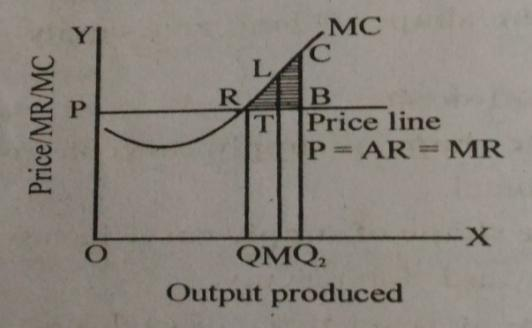As shown in the diagram that price is less than marginal cost at the production level OM or OQ2. So, it is proved that profit maximization point neither be M nor Q2. Suppose the production has been reduced from OQ2 level to OM level. As a result, the reduction of total cost is Q2 MLC, which is larger than the reduction of total revenue that is Q2MTB. The shaded area BTLC is the loss portion of the firm. Therefore, the firms profit maximizing production level can neither be OM nor OQ2. It means that the market price cannot be less than the marginal cost. Thus, at the profit maximizing production level of OQ, price is equal to MC.
42. Draw the average revenue and marginal revenue curve of the perfectly competitive market.
Ans: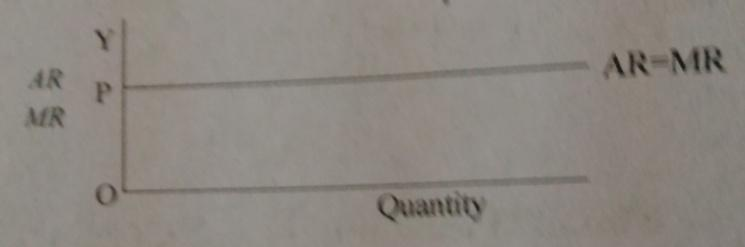43. Explain the concept of market equilibrium with the help of demand and supply. Or Explain how the shifting of the demand curve for a commodity affects the equilibrium price and output. 2015, 2016
Ans: When quantity demand and quantity supply of a commodity are equal then a market gets an equilibrium position. At that equilibrium position, the amount demanded at this price is equal to the amount supplied. That means total supply of the good that all the sellers want to sell is exactly equal to the total supply which all the consumers. It is determined at a point where demand and supply curves intersect each other.
In the perfectly competitive market determines equilibrium price and quantity by the interaction of demand and supply. The impact of simultaneous shift of demand and supply on equilibrium price and quantity is depicted by a schedule. Simultaneous shift of demand and supply may be four types that are as follows:
1. Both the demand curve and supply curve shift rightwards.
2. Both the demand curve and supply curve shift leftwards.
3. Demand curve shifts rightward and supply curve shifts leftward.
4. Demand curve shifts leftward and supply curve shift rightward.
 Shift of Demand Shift of Supply Quantity Price Leftward Rightward Rightward Leftward Leftward Rightward Leftward Rightward Decrease Increase Rise/fall change in Rise/fall change in Rise/fall remain constant Rise/fall remain constant Rise Fall
The following schedule diagram illustrates the determination of market equilibrium.
 Price (Rs.) Demand Supply 6 5 4 3 2 1 50 100 200 300 400 500 600 500 400 300 200 100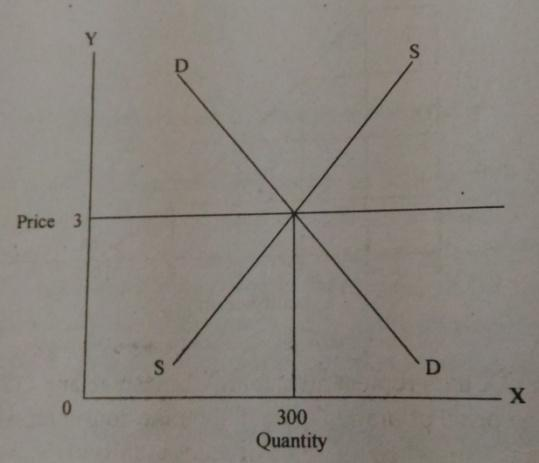The above table and diagram show that the equilibrium is attained at that point E, where at price Rs. 3, the quantity demand is 300 and quantity supply is also 300 intersect each other and the firm gets equilibrium position.

44. Write the assumptions of market equilibrium.
Ans: Following are the assumptions of market equilibrium:
1. The taste and preference of consumers remain constant.
2. The income of the consumers remains constant.
3. Technology of production, the market size remains constant.
4. The price of factors of production remains constant.

45. Explain the concept of revenue curve under perfect competition.
Ans: Revenue Curve under Perfect competition: Perfect competition is the term applied to a situation in which the individual buyer or seller (firm) represent such a small share of the total business transacted in the market that he exerts no perceptible influence on the price of the commodity in which he deals. Thus, in perfect competition an individual firm is price taker, because the price is determined by the collective forces of market demand and supply which are not influenced by the individual. When price is the same for all units of a commodity, naturally AR (Price) will be equal to MR i.e., AR = MR. The revenue schedule for a competitive firm is shown in the table 5.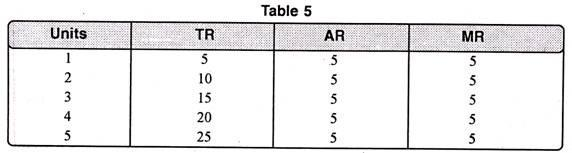In table 5 we find that as output increases, AR remains the same i.e. Rs. 5. Total revenue increases but at a constant rate. Marginal revenue is also constant i.e. Rs. 5 and is equal to AR. Thus TR = AR x Q
Also TR = MR x Q [Since AR = MR]. In figure 8, on the X-axis, we take quantity whereas on Y-axis, we take revenue. At price OP, the seller can sell any amount of the commodity. In this case the average revenue curve is the horizontal line. The Marginal Revenue curve coincides with the Average Revenue. It is because additional units are sold at the same price as before. In that case AR = MR. A noteworthy point is that OP price is determined by demand and supply of industry. The firm only follows, (see figure below):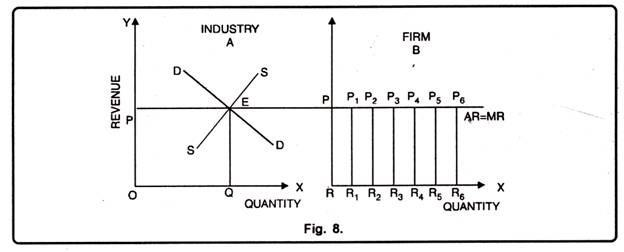46. Draw and explain the price line of a firm under perfect competitive market with a suitable diagram.  [AHSEC 2012]
Ans. Price line is a horizontal line that represents the market price for a perfectly competitive firm. It also signifies the demand curve faced by the firm. This is shown in the diagram.

In the diagram P = AR = MR represents the price line of a firm under perfect competition. For a perfectly competitive firm, price is given. It remains the same at all output levels.